#### 期刊菜单

Band Structure and Quantum Phase Transition of Graphene/h-BN Heterojunction under Local Potential Control
DOI: 10.12677/CMP.2022.113007, PDF, HTML, XML, 下载: 127  浏览: 247  国家自然科学基金支持

Abstract: The band structure and quantum phase transition of graphene/hexagonal boron nitride heterojunction (h-BN) under local potentials are studied by the tight-binding method. The result shows that the graphene layer is in the quantum spin Hall state and the h-BN layer is in the insulating state when the intrinsic spin-orbit coupling strength of the graphene layer is given. As the local potential of the graphene layer increases, the system will change from a quantum spin Hall state to a semiconductor state. New gapless edge states can be generated by tuning the local potential of the h-BN layer, so that the quantum spin Hall state of the graphene layer becomes the quantum spin Hall state composed of the edge states within and between layers.

1. 介绍

2. 模型与方法

${H}_{MLG}=-{t}_{1}\underset{〈i,j〉,\alpha }{\sum }{C}_{i\alpha }^{†}{C}_{j\alpha }+i\lambda \underset{〈〈i,j〉〉,\alpha \beta }{\sum }{\upsilon }_{ij}{C}_{i\alpha }^{†}{\left({S}_{z}\right)}_{\alpha \beta }{C}_{j\beta }+\underset{i,\alpha }{\sum }{V}_{i}{C}_{i\alpha }^{†}{C}_{i\alpha }$ (1)

${H}_{h\text{-}BN}=-{t}_{2}\underset{〈i,j〉\alpha }{\sum }{C}_{i\alpha }^{†}{C}_{j\alpha }+\underset{i,\alpha }{\sum }{E}_{i}{C}_{i\alpha }^{†}{C}_{i\alpha }+\underset{i,\alpha }{\sum }{V}_{i}{C}_{i\alpha }^{†}{C}_{i\alpha }$ (2)

Bernal堆叠石墨烯/h-BN异质结的哈密顿量为：

$H={H}_{MLB}+{H}_{h\text{-}BN}+{t}_{\perp }\underset{i\in T,j\in B,\alpha }{\sum }\left({C}_{i\alpha }^{†}{C}_{j\alpha }+{C}_{j\alpha }^{†}{C}_{i\alpha }\right)$ (3)

${C}_{\alpha \beta }=\frac{1}{2\pi }\underset{n}{\sum }{\int }_{BZ}\text{d}{k}_{x}\text{d}{k}_{y}{\left({\Omega }_{xy}\right)}_{\alpha \beta }$ (4)

${\Omega }_{xy}=-\underset{{n}^{\prime }\ne n}{\sum }\frac{2\mathrm{Im}〈{\Psi }_{nk}|{v}_{x}|{\Psi }_{{n}^{\prime }k}〉〈{\Psi }_{{n}^{\prime }k}|{v}_{y}|{\Psi }_{nk}〉}{{\omega }_{n}-{\omega }_{{n}^{\prime }}}$ (5)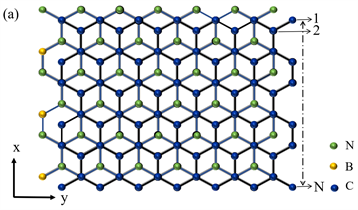Figure 1. (a) Schematic diagram of Bernal stacking zigzag-edged GBNNR. The bottom layer is h-BN layer and the top layer is graphene layer. y is the periodic direction and the x direction contains N = 80 sites. Green, yellow and blue solid circles represent N, B and C atoms, respectively; (b) Schematic diagram of graphene/h-BN heterojunction under local potentials.V1 and V2 are applied in the upper and lower half regions of the graphene layer respectively, and V3 and V4 are applied in the upper and lower half regions of the h-BN layer respectively

3. 结果与讨论Table 1. Tight-binding parameters of graphene/h-BN heterojunctions calculated by density functional theory  (Unit of Energy: eV)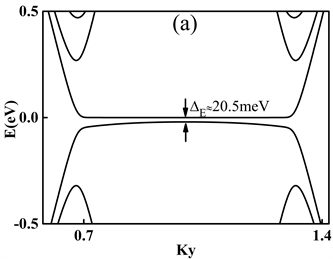Figure 2. (a) Band structure of GBNNR with zero local potential and ISOC; (b) Band gap of GBNNR ΔE as a function of V1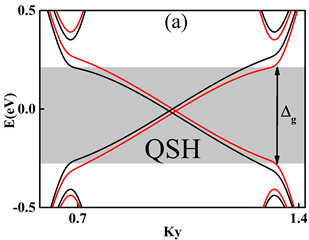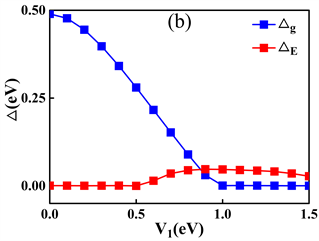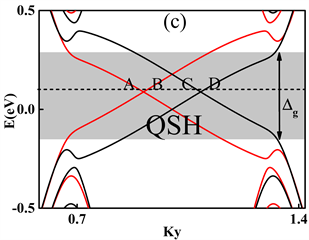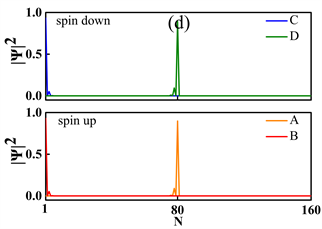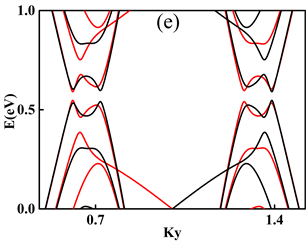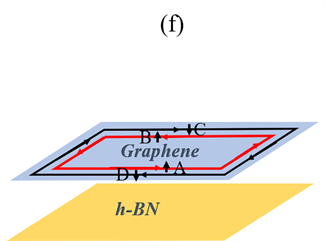Figure 3. Left column is the band structure of GBNNR. The red and black curves represent the spin-up and spin-down energy bands respectively. The parameters are: λ = 0.02t1, (a) V1 = 0 eV; (c) V1 = 0.2 eV; (e) V1 = 1.2 eV. (b) The blue curve is the bandgap of the heterojunction under periodic boundary conditions, i.e. the bulk gap; the red curve is the GBNNR bandgap. (d) and (f) are the schematic diagrams of the probability distributions of edge states and the edge state propagation in (c), respectively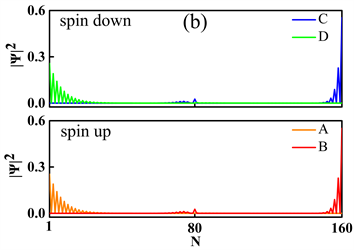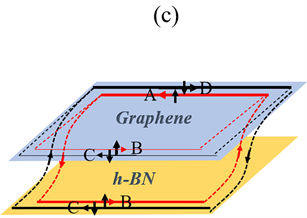Figure 4. (a) Band structure of GBNNR with λ = 0.02 t1, V4 = −3.665 eV. The red and black curves represent the spin-up and spin-down energy bands respectively. (b) and (c) are schematic diagrams of the probability distributions of edge states and the edge state propagation in (a), respectively

4. 结论

NOTES



*通讯作者。

  Sarma, S.D., Adam, S., Hwang, E.H., et al. (2011) Electronic Transport in Two-Dimensional Graphene. Reviews of Modern Physics, 63, 407-470. https://doi.org/10.1103/RevModPhys.83.407  Rutherglen, C., Jain, D. and Burke, P. (2009) Nanotube Electronics for Radiofrequency Applications. Nature Nanotechnology, 4, 811-819. https://doi.org/10.1038/nnano.2009.355  Moon, P. and Koshino, M. (2014) Electronic Properties of Graphene/Hexagonal-Boron-Nitride Moiré Superlattice. Physical Review B, 90, Article ID: 155406. https://doi.org/10.1103/PhysRevB.90.155406  N’Diaye, A.T., Bleikamp, S., Feibelman, P.J., et al. (2006) Two-Dimensional Ir Cluster Lattice on a Graphene Moiré on Ir(111). Physical Review Letters, 97, Article ID: 215501. https://doi.org/10.1103/PhysRevLett.97.215501  Varchon, F., Feng, R., Hass, J., et al. (2007) Electronic Structure of Epitaxial Graphene Layers on SiC: Effect of the Substrate. Physical Review Letters, 99, Article ID: 126805. https://doi.org/10.1103/PhysRevLett.99.126805  Sachs, B., Wehling, T.O., Katsnelson, M.I., et al. (2011) Adhesion and Electronic Structure of Graphene on Hexagonal Boron Nitride Substrates. Physical Review B, 84, Article ID: 195414. https://doi.org/10.1103/PhysRevB.84.195414  Slotman, G.J., Wijk, M.V., Zhao, P.L., et al. (2015) Effect of Structural Relaxation on the Electronic Structure of Graphene on Hexagonal Boron Nitride. Physical Review Letters, 115, Article ID: 186801. https://doi.org/10.1103/PhysRevLett.115.186801  Fan, Y., Zhao, M., Wang, Z., et al. (2011) Tunable Electronic Structures of Graphene/Boron Nitride Heterobilayers. Applied Physics Letters, 98, Article ID: 083103. https://doi.org/10.1063/1.3556640  Sun, J., Xu, L. and Zhang, J. (2020) Electronic Structure and Transport Properties of Graphene/h-BN Controlled by Boundary Potential and Magnetic Field. Modern Physics Letters B, 34, Arti-cle ID: 2050180. https://doi.org/10.1142/S0217984920501808  Li, X., Xu, L. and Zhang, J. (2020) Band Structure and Transport Property of Graphene/h-BN Heterostructure under Local Potentials. Chinese Journal of Physics (Taipei), 65, 75-81. https://doi.org/10.1016/j.cjph.2020.02.011  Kane, C.L. and Mele, E.J. (2004) Quantum Spin Hall Effect in Graphene. Physical Review Letters, 95, Article ID: 226801. https://doi.org/10.1103/PhysRevLett.95.226801  Kane, C.L. and Mele, E.J. (2005) Z2 Topological Order and the Quantum Spin Hall Effect. Physical Review Letters, 95, Article ID: 146802. https://doi.org/10.1103/PhysRevLett.95.146802  Sheng, D.N., Weng, Z.Y., Sheng, L., et al. (2006) Quantum Spin Hall Effect and Topologically Invariant Chern Numbers. Physical Review Letters, 97, Article ID: 036808. https://doi.org/10.1103/PhysRevLett.97.036808  Sheng, L., Sheng, D.N., Ting, C.S., et al. (2005) Nondissipative Spin Hall Effect via Quantized Edge Transport. Physical Review Letters, 95, Article ID: 136602. https://doi.org/10.1103/PhysRevLett.95.136602  Prodan, E. (2009) Robustness of the Spin-Chern Number. Physical Review, 80, Article ID: 125327. https://doi.org/10.1103/PhysRevB.80.125327  Sheng, L., Sheng, D.N. and Ting, C.S. (2005) Spin-Hall Effect in Two-Dimensional Electron Systems with Rashba Spin-Orbit Coupling and Disorder. Physical Review Letters, 94, Ar-ticle ID: 016602. https://doi.org/10.1103/PhysRevLett.94.016602  Andrei Bernevig, B. and Zhang, S.-C. (2006) Quantum Spin Hall Effect. Physical Review Letters, 96, Article ID: 106802. https://doi.org/10.1103/PhysRevLett.96.106802  Xu, L., Zhou, Y. and Gong, C.D. (2013) Topological Phase Transition Induced by Spin-Orbit Coupling in Bilayer Graphene. Journal of Physics Condensed Matter, 25, Article ID: 335503. https://doi.org/10.1088/0953-8984/25/33/335503  Ren, Y., Qiao, Z. and Niu, Q. (2016) topological Phases in Two-Dimensional Materials: A Review. Reports on Progress in Physics Physical Society, 79, Article ID: 066501. https://doi.org/10.1088/0034-4885/79/6/066501  Chen, T.W., Xiao, Z.R., Chiou, D.W., et al. (2011) High Chern Number Quantum Anomalous Hall Phases in Single-Layer Graphene with Haldane Orbital Coupling. Physical Review B, 84, Article ID: 165453. https://doi.org/10.1103/PhysRevB.84.165453  Wang, E., Lu, X., Ding, S., Yao, W., Yan, M., Wan, G., et al. (2016) Gaps Induced by Inversion Symmetry Breaking and Second-Generation Dirac Cones in Graphene/Hexagonal Boron Nitride. Nature Physics, 12, 1111-1115. https://doi.org/10.1038/nphys3856  Yang, W., Chen, G., Shi, Z., Liu, C.-C., Zhang, L., Xie, G., et al. (2013) Epitaxial Growth of Single-Domain Graphene on Hexagonal Boron Nitride. Nature Materials, 12, 792-797. https://doi.org/10.1038/nmat3695  Gorbachev, R.V., Song, J.C.W., Yu, G.L., Kretinin, A.V., Withers, F., Cao, Y., et al. (2014) Detecting Topological Currents in Graphene Superlattices. Science, 346, 448-451. https://doi.org/10.1126/science.1254966  Den Nijs, M. (1984) Quantized Hall Conductance in a Two Dimen-sional Periodic Potential. Physica A: Statistical Mechanics and Its Applications, 124, 199-210. https://doi.org/10.1016/0378-4371(84)90239-5  Kohmoto, M. (1985) Topological Invariant and the Quantiza-tion of the Hall Conductance. Annals of Physics, 160, 343-354. https://doi.org/10.1016/0003-4916(85)90148-4  Hatsugai, Y. (1993) Chern Number and Edge States in the Integer Quantum Hall Effect. Physical Review Letters, 71, 3697-3700. https://doi.org/10.1103/PhysRevLett.71.3697  Chang, M.C. and Niu, Q. (1995) Berry Phase, Hyperorbits, and the Hofstadter Spectrum. Physical Review Letters, 75, 1348-1351. https://doi.org/10.1103/PhysRevLett.75.1348  Slawinska, J., Zasada, I., Kosinski, P., et al. (2010) Reversible Modifications of Linear Dispersion: Graphene between Boron Nitride Monolayers. Physical Review B: Condensed Matter, 82, Article ID: 085431. https://doi.org/10.1103/PhysRevB.82.085431